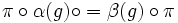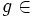# Using group actions and representations to solve the extensible automorphisms problem

(diff) ← Older revision | Latest revision (diff) | Newer revision → (diff)
This is a survey article related to:Extensible automorphisms problem
View other survey articles about Extensible automorphisms problem

An automorphism$\sigma$ of a group$G$ is termed extensible if, for any group$H$ containing$G$, there is an automorphism$\sigma'$ of$H$ whose restriction to$G$ equals$\sigma$.

The extensible automorphisms problem asks for a characterization of all the extensible automorphisms of any group. Clearly, any inner automorphism is extensible. It turns out that the converse is also true: every extensible automorphism is inner. However, this is not obvious at all. This page describes some partial approaches that don't come close to establishing that extensible automorphisms are inner, but does constrain them considerably by showing that they imply some properties implied by being inner.

Two main methods of attack for the problem are the use of group actions on sets and the use of linear representations, i.e., group actions on vector spaces. This article discusses a general common idea behind these methods, and the reasons behind the partial success as well as the limitations of such approaches.

## The main steps of the approach

### Step one: Reduce extensibility of the automorphism to being extensible to an inner automorphism with respect to that kind of action

Suppose$S$ is a structure with an automorphism group$A = \operatorname{Aut}(S)$. (The automorphisms are the automorphisms that preserve certain specified structural properties of$S$, these may be all permutations, linear maps, algebra automorphisms, self-homeomorphisms, isometries, or other things). A representation of$G$ on$S$ is a homomorphism$\alpha: G \to \operatorname{Aut}(S)$. Two representations$\alpha:G \to \operatorname{Aut}(S)$ and$\beta:G \to \operatorname{Aut}(T)$ are equivalent if there is an isomorphism$\pi:S \to T$ such that, for any$g \in G$:$\pi \circ \alpha(g) \circ = \beta(g) \circ \pi$.

In particular, if$S = T$, two representations of$G$ on$S$ are equivalent if there is an automorphism of$S$ such that conjugation by that automorphism in$\operatorname{Aut}(S)$ sends one homomorphism to the other.

The first step is to use the fact that an automorphism is extensible to deduce that for certain kinds of representations, it can be extended to an inner automorphism of the automorphism group. In other words, we would like to say that if$\sigma$ is an extensible automorphism of$G$, then for any representation$\alpha$ of$G$ on a structure$S$,$\alpha$ and$\alpha \circ \sigma$ are equivalent, i.e., there exists an element of$\operatorname{Aut}(S)$ that conjugates$\alpha(g)$ to$\alpha(\sigma(g))$ for all$g \in$.

This is tricky, and the way it is achieved is different in the linear representations and group actions case:

### Step two: relate extensibility to inner automorphisms for every representation to some group-theoretic constraint on the automorphism

With the completion of step one, we have shown that if$\sigma$ is an extensible automorphism of$G$, then$\sigma$ can be pushed forward to an inner automorphism of$\operatorname{Aut}(S)$ for any homomorphism from$G$ to$\operatorname{Aut}(S)$. The next step is to relate the property of being pushforwardable to an inner automorphism of$\operatorname{Aut}(S)$ to some intrinsic group-theoretic constraint.

The approach for doing this depends on the specific case. In the case of linear representations, we use conjugacy class-representation duality, that is valid over a sufficiently large field, to show that an automorphism is linearly pushforwardable for all finite-dimensional representations over such a field if and only if it is class-preserving. This duality simply rests on the facts that a representation over a sufficiently large field is completely determined by its character, and any two distinct conjugacy classes can be separated by the character of some representation.

Note that although there is a conjugacy class-representation duality, there is no natural bijection between the conjugacy classes and representations, so transforming the problem from linearly pushforwardability to class-preserving requires us to consider the collection of all irreducible representations.

In the case of permutation representations, we use the fundamental theorem of group actions, that naturally relates conjugacy classes of subgroups with transitive group actions. Using this relation, we can show that an automorphism can be pushed forward for every permutation representation if and only if it is a subgroup-conjugating automorphism, i.e., it sends every subgroup to a conjugate subgroup.